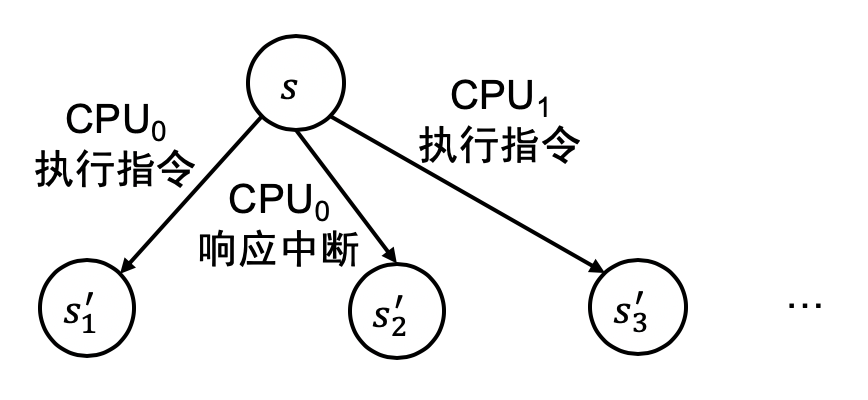# 并发控制：互斥 (2)

## 1. 解决自旋造成的浪费

### 1.1. 自旋锁和浪费问题

while (xchg(&locked, 1)) {
// 获得锁失败，需要再次尝试
}


## 2. 操作系统中的互斥

### 2.1. 计算机硬件系统的状态机模型

#### 2.1.1. “计算机系统” 的状态

• -nographic 不启动图形界面 (所以做操作系统实验其实并不需要任何图形界面……)
• -S 使 QEMU 在 CPU Reset 之后暂停不再执行指令

(qemu) info registers
EAX=00000000 EBX=00000000 ECX=00000000 EDX=00000663
ESI=00000000 EDI=00000000 EBP=00000000 ESP=00000000
EIP=0000fff0 EFL=00000002 [-------] CPL=0 II=0 A20=1 SMM=0 HLT=0
ES =0000 00000000 0000ffff 00009300
CS =f000 ffff0000 0000ffff 00009b00
SS =0000 00000000 0000ffff 00009300
DS =0000 00000000 0000ffff 00009300
FS =0000 00000000 0000ffff 00009300
GS =0000 00000000 0000ffff 00009300
LDT=0000 00000000 0000ffff 00008200
TR =0000 00000000 0000ffff 00008b00
GDT=     00000000 0000ffff
IDT=     00000000 0000ffff
CR0=60000010 CR2=00000000 CR3=00000000 CR4=00000000
DR0=0000000000000000 DR1=0000000000000000 DR2=0000000000000000 DR3=0000000000000000
DR6=00000000ffff0ff0 DR7=0000000000000400
EFER=0000000000000000
FCW=037f FSW=0000 [ST=0] FTW=00 MXCSR=00001f80
FPR0=0000000000000000 0000 FPR1=0000000000000000 0000
FPR2=0000000000000000 0000 FPR3=0000000000000000 0000
FPR4=0000000000000000 0000 FPR5=0000000000000000 0000
FPR6=0000000000000000 0000 FPR7=0000000000000000 0000
XMM00=00000000000000000000000000000000 XMM01=00000000000000000000000000000000
XMM02=00000000000000000000000000000000 XMM03=00000000000000000000000000000000
XMM04=00000000000000000000000000000000 XMM05=00000000000000000000000000000000
XMM06=00000000000000000000000000000000 XMM07=00000000000000000000000000000000


RAX=00000000000048af RBX=0000000000103520 RCX=0000000000000001 RDX=00000000000003f8
RSI=0000000000000001 RDI=0000000000000000 RBP=0000000000103527 RSP=0000000000106f30
R8 =0000000000000000 R9 =0000000000000000 R10=0000000000000000 R11=0000000000000000
R12=0000000000000000 R13=0000000000000000 R14=0000000000000000 R15=0000000000000000
RIP=00000000001001d0 RFL=00000283 [--S---C] CPL=0 II=0 A20=1 SMM=0 HLT=0
ES =0000 0000000000000000 00000000 00000000
CS =0008 0000000000000000 00000000 00209a00 DPL=0 CS64 [-R-]
SS =0000 0000000000000000 ffffffff 00c09300 DPL=0 DS   [-WA]
DS =0000 0000000000000000 00000000 00000000
FS =0000 0000000000000000 00000000 00000000
GS =0000 0000000000000000 00000000 00000000
LDT=0000 0000000000000000 0000ffff 00008200 DPL=0 LDT
TR =0028 0000000000108060 00000067 00408900 DPL=0 TSS64-avl
GDT=     0000000000108028 00000038
IDT=     0000000000106fc0 00001000
CR0=80000011 CR2=0000000000000000 CR3=0000000000001000 CR4=00000020
DR0=0000000000000000 DR1=0000000000000000 DR2=0000000000000000 DR3=0000000000000000
DR6=00000000ffff0ff0 DR7=0000000000000400
EFER=0000000000000500
FCW=037f FSW=0000 [ST=0] FTW=00 MXCSR=00001f80
FPR0=0000000000000000 0000 FPR1=0000000000000000 0000
FPR2=0000000000000000 0000 FPR3=0000000000000000 0000
FPR4=0000000000000000 0000 FPR5=0000000000000000 0000
FPR6=0000000000000000 0000 FPR7=0000000000000000 0000
XMM00=00000000000000000000000000000000 XMM01=00000000000000000000000000000000
XMM02=00000000000000000000000000000000 XMM03=00000000000000000000000000000000
XMM04=00000000000000000000000000000000 XMM05=00000000000000000000000000000000
XMM06=00000000000000000000000000000000 XMM07=00000000000000000000000000000000
XMM08=00000000000000000000000000000000 XMM09=00000000000000000000000000000000
XMM10=00000000000000000000000000000000 XMM11=00000000000000000000000000000000
XMM12=00000000000000000000000000000000 XMM13=00000000000000000000000000000000
XMM14=00000000000000000000000000000000 XMM15=00000000000000000000000000000000


#### 2.1.2. “计算机系统” 作为状态机的执行

• 如果运行在 ring 3，切换到 TSS 中预设的堆栈地址 (%ss, %rsp)；
• 将中断前的若干寄存器保存到堆栈上：%ss, %rsp, %cs, %rip, %rflags
• 跳转到 IDT 中指定的入口地址执行。

AbstractMachine 对中断的底层行为进行了封装，在中断到来后会寄存器现场 (Context) 到堆栈上，最后调用注册的 callback (这里建议你再次阅读 AbstractMachine 文档)。在用状态机模型分析直接运行在计算机硬件上的程序 (例如操作系统) 时要格外小心，因为多处理器系统的状态机模型上，状态机的每一步都有很大的不确定性：

• 可以选择系统中任意一个处理器执行一条指令；
• 任意一个处理器在中断打开时都可以响应系统中的中断。## 2.2. 在操作系统中实现互斥

int locked = 0;
void lock()   { while (atomic_xchg(&locked, 1)); }
void unlock() { atomic_xchg(&locked, 0); }


### 2.2.1. 单处理器：关中断 = Stop the World

void lock(lock_t *lk) {
cli();
}
void unlock(lock_t *lk) {
sti();
}


#### 思考题：何时开中断？

lock(&lk1);
lock(&lk2);
unlock(&lk2); // 1
unlock(&lk1); // 2


### 2.2.2. 多处理器：Stop the World + 自旋

void lock(lock_t *lk) {
cli();
while (atomic_xchg(&lk->locked, 1)) ;
}
void unlock(lock_t *lk) {
atomic_xchg(&lk->locked, 0);
sti();
}


#### 思考题：操作顺序

cli 还是先自旋？先释放锁还是先 sti？注意我们必须保证在获得锁期间处理器不会被中断。在 cli() 返回前的任意时刻，处理器都会被中断；在 sti() 后的一瞬间，处理器也可能被中断。

void case1() {
lock(&lk):
unlock(&lk);
// 上锁/解锁前后IF标志位不变，不得随意打开中断
}

void case2() {
assert((read_eflags() & FL_IF) != 0); // 初始时中断打开
lock(&lk1);
lock(&lk2);
unlock(&lk2);
assert((read_eflags() & FL_IF) == 0); // 持有锁时不得开中断
unlock(&lk1);
assert((read_eflags() & FL_IF) != 0); // 在释放所有锁后开中断
}


#### 善用断言 (assertions)

xv6 的 spinlock 实现是这方面的典范。这也是我们强烈推荐 xv6 代码的原因。